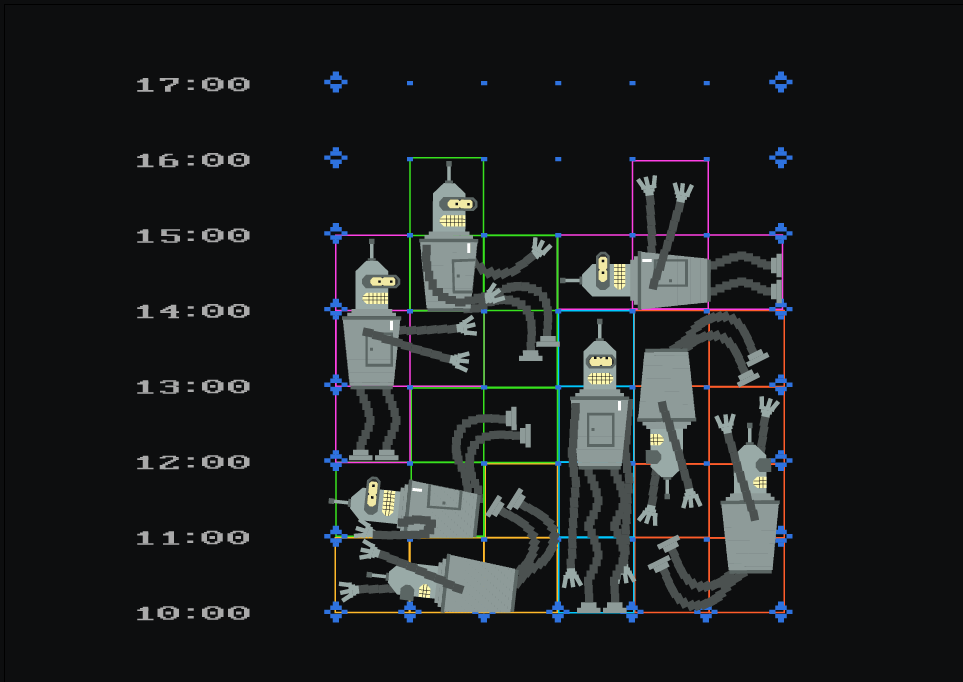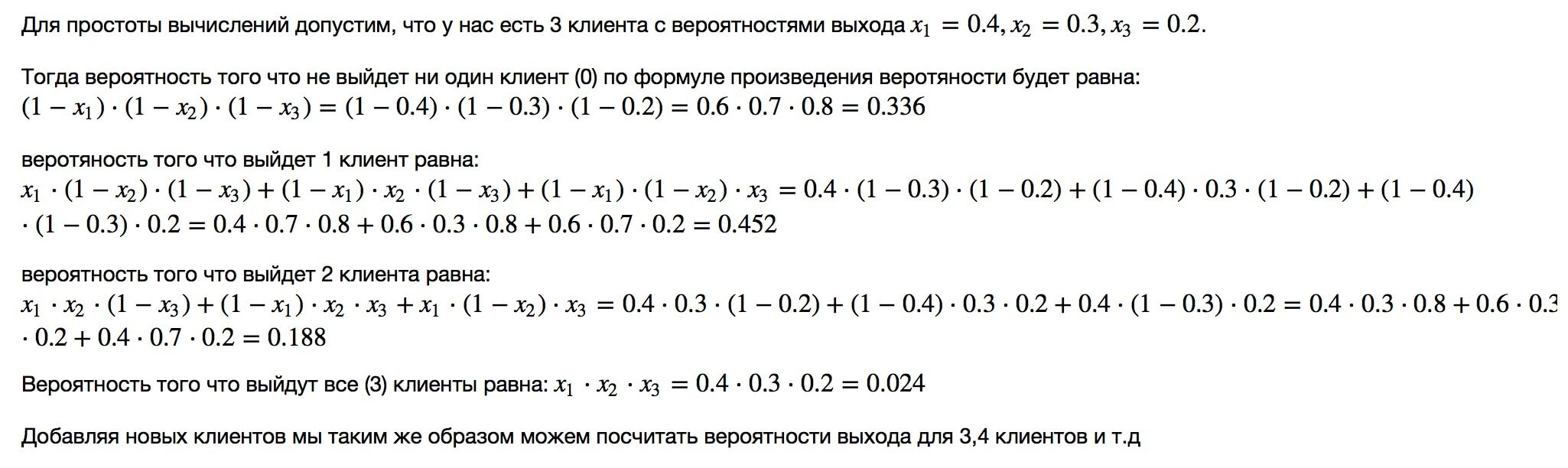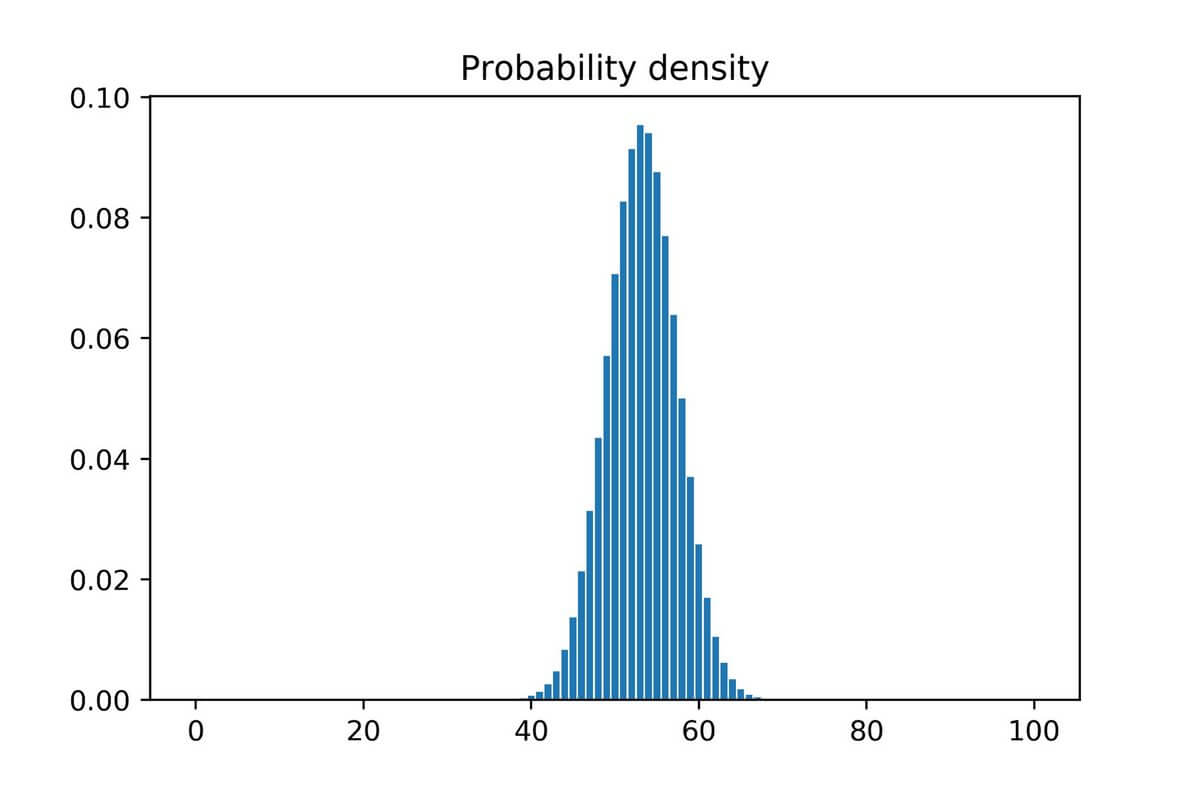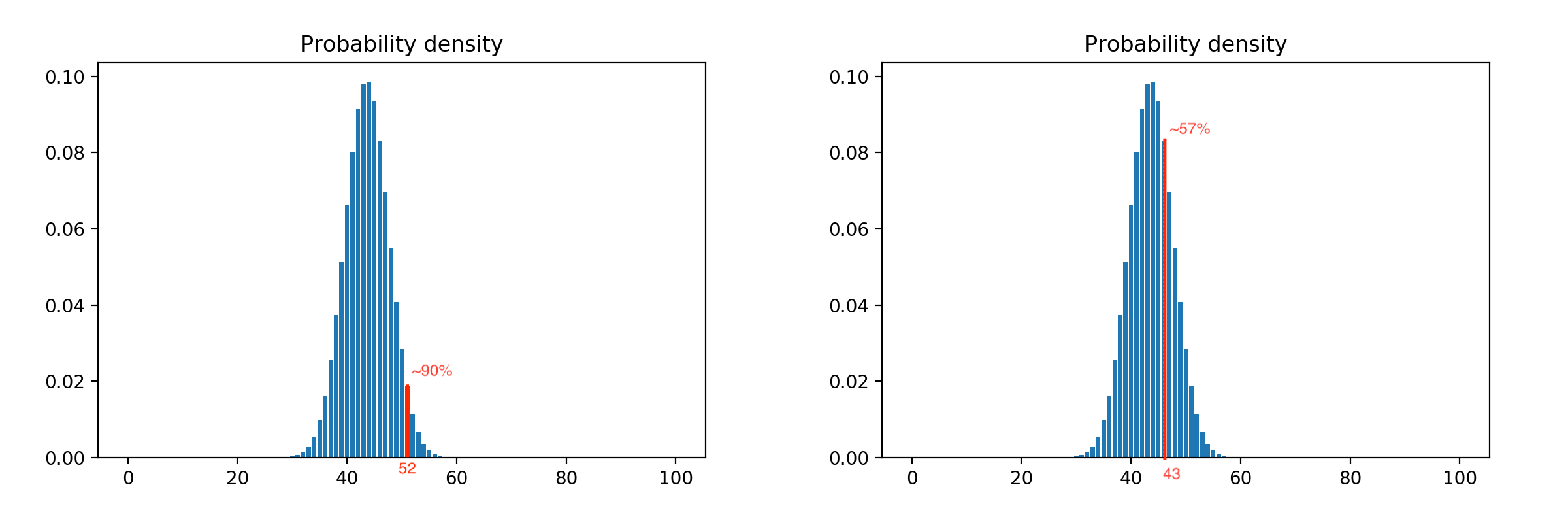How we halved the cost of an introductory lesson by looking at a solution with airlinesA free introductory lesson is a feature of Skyeng School. A potential student can get acquainted with the platform on it, check their level of English, finally, just have fun. For the school, the introductory lesson is part of the sales funnel, which should be followed by the first payment. He is conducted by the methodologist of the introductory lesson – a special person combining the teacher and sales person, his time is paid regardless of whether the client bought the first package or not, and whether he came to the class at all. Non-appearance is a very frequent phenomenon, because of which the price of a lesson becomes too high.

In this article, we will describe how, with the help of an analytical model and airline experience, we were able to reduce the cost of an introductory lesson by almost half.

Skyeng sales funnel consists of five steps: registration on the site, the call to the first sales line with a record for the introductory lesson, the introductory lesson, the call to the second sales line, payment for the first package. Previously, after the first phone call, we set a lesson time for a particular methodologist of the introductory lesson, who was waiting for the student at that time. If a person enrolled and did not come, the methodologist wastes his time, and the school – money to pay for this time. Non-attendance occurs on average in half the cases; one third of customers buy the first package after the introductory lesson. Thus, the conversion from the entry for the introductory lesson to the payment is only 0.15. A successful (converted into payment) introductory lesson in the old scheme cost us 4,000 rubles, and we had to do something about it.

You can simply refuse it, but in this case, the final conversion from the lead into payment will drop dramatically, which does not suit us. We'll have to look for another solution, build models, count and experiment.

First pancake

We turned to the experience of airlines, specifically to the practice of overbooking. Carriers know that 100% of passengers who bought a ticket are rarely a flight, and use this by selling more tickets than seats in an airplane. If suddenly all the passengers arrive at the landing, you can find among them volunteers who are ready to fly on the next flight for a bun. Airlines thus increase their profits, and we can reduce costs by a similar method.

So: we refuse to write to a specific person, create a pool of methodologists of the introductory lesson, scatter applications between them on the basis that half will not appear. And if more has come, we offer to sign up for another day. We launched such an MVP into the test and immediately realized that we did everything wrong.

Half of those who go to the introductory lesson are statistics, in reality the share fluctuates greatly depending on the time, day, channel, from which the person came. At the same time, more than 80% of potential students in response to the proposal to transfer the lesson or immediately fall off, or do not come on the second record. All this could lead to the fact that in bad days we would lose up to a third of clients. The test turned and went to do everything rationally.

Model, forecasts, polynomials

First of all, it was necessary to find out what the share of those going to the introductory lesson depends on. The first observation is that it depends on the marketing channel from which the person came. We divide these channels in terms of conversion into payment for “hot”, where the conversion is higher, “warm” and “cold”, where it is lower; It turned out that the “channel temperature” affects the conversion to the output to the introductory lesson in about the same way.

Continuing the aviation analogy, we made different “check-in counters” for leads from different channels, placing them with coefficients corresponding to the historical probability of the release of this channel: 0.8, 0.4 and 0.2. We allocate more Methodists for the “hot” channels, and less for the “cold” ones. It worked better, but still on bad days there were more than 20% of the “departures” (situations where more clients turned to the introductory lesson than there were free methodologists). We tried to increase the coefficients by adding a margin of 0.1: on the one hand, the more methodologists we derive, the less we lose customers, on the other – the costs of conducting introductory lessons increase.

From these observations, the second MVP has grown. For each enrolled, we build a forecast of the probability of his exit to the introductory lesson. We make a joint probability distribution and confidence interval with a confidence level of 95%. For rare cases, when more clients come out than planned, we keep a reserve pool of methodologists – teachers who are currently engaged in non-urgent work like checking essays.

To calculate the forecast for a particular student, we built a statistical model based on our historical data and taking into account several factors: channel, region, child / adult, private / corporate client, the time from recording to the introductory lesson.

The model uses the following concepts:

• slot: date and time of introductory lesson;
• correction factor: probability of abnormal exit on this day and hour;
• application weight: admissible probability of exit of the given client;
• departure: unserved application (client left, all methodologists are busy);
• simple methodologist: it turned out less than predicted, people are idle;
• restriction:% in the confidence interval, after reaching which the model prohibits adding orders to the slot.

In each slot there are N Methodists, and the slot itself has correction factor k (with base 100). The number of methodologists available for the model is defined as round (N * k / 100). When the application appears, the model determines its weightIt looks at the sum of such weights already in the slot, and determines the slot as available if, as a result of the addition of this application, the sum of the weights of applications in the slot will not exceed the number of methodologists. The metrics for estimating the model are: the share of departures (to be minimized), the loading of the slot (maximized), the waiting time for the introductory lesson by the client (minimized). Variable parameters of the model are application weight and restriction.

For the forecast of how many customers will be released, the formula of the product of probabilities was used:Considering all possible combinations of outputs, we get a very close to natural distribution of probabilities. The distribution for one hundred customers looks like this:By applying a confidence interval to it, we can adjust the aggressiveness of the model. For example, shifting the restriction to the left increases it, i.e. we release more clients with the same number of methodologists, and the shift to the right reduces, because restriction works earlier. Example with 90% and 57% restrictions:In addition, the aggressiveness of the model can be adjusted by a correction factor: reducing it reduces, increasing – raises. This is useful when we know that on a particular day / hour, some external factors can make the abnormality of the anomalous.

The formula with the multiplication of probabilities worked well in tests, but it was rather computationally hard, so we rewrote it with polynomials:The disadvantages of the model include:

• due to the fact that it relies on historical data, it reacts poorly to abrupt changes in the output;
• if the methodologist had a force majeure and he falls out of the slot, this is almost a guaranteed departure, managers need to urgently reassign the lesson;
• if the dynamic marking of the “heat” of the channels falls, the model incorrectly estimates the probability of the client’s exit.

As a result of using this model, we received up to 45% cost savings on the introductory lesson with minimal customer losses.

Why not machine learning?

Because the statistical model works so well, and instead of improving the accuracy of the existing forecast with the help of ML, it is more profitable to direct the forces of ML developers to other tasks.

For example, we are developing a potential client scoring system, remotely similar to a banking one. Banks by scoring determine the probability of repayment of the loan, and we can determine the probability of the first payment. If it is very low, there is no need to spend resources on organizing an introductory lesson; if, on the contrary, it is very high, you can immediately send the client to the payment page.

But this story is for another time.